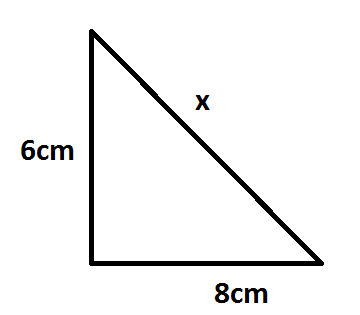Find the unknown length x in the following figure:

# Find the unknown length x in the following figure:1. A
7
2. B
8
3. C
9
4. D
10

Fill Out the Form for Expert Academic Guidance!l

+91

Live ClassesBooksTest SeriesSelf Learning

Verify OTP Code (required)

### Solution:

${a}^{2}+{b}^{2}={c}^{2}$ …(1)
Now according to this question we have, a=6, b=8, c=x
Substitute the values in equation (1), we have,
${6}^{2}+{8}^{2}={x}^{2}=36+64=100={10}^{2}$
$⇒x=10$

## Related content

 Area of Square Area of Isosceles Triangle Pythagoras Theorem Triangle Formula Perimeter of Triangle Formula Area Formulae Volume of Cone Formula Matrices and Determinants_mathematics Critical Points Solved Examples Type of relations_mathematics+91

Live ClassesBooksTest SeriesSelf Learning

Verify OTP Code (required)